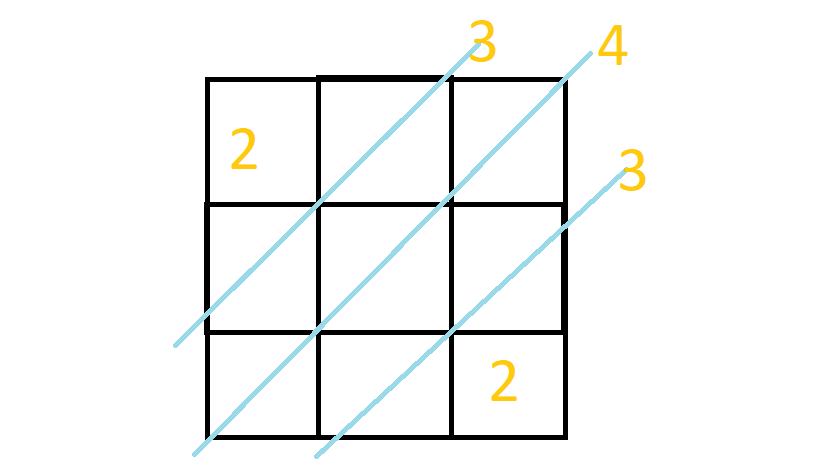### 珂朵莉

（快去看！出门左转樱x动漫！）$$\sum^k_{i=1}{c^2_i}$$

P1081

### 题目简述

A和B自左向右开车旅行（出发点可以变）

A、B的开车风格：

1.A会前往右边距他第二近的城市
2.B会前往右边最近的城市
3.A和B开过的总里程不超过$X$

1.给定一个$X$ 求出从哪个城市出发 $A$的路程/$B$的路程 最大

2.给定$X_i$和出发城市$S_i$ 求$A$和$B$分别开的距离数

## 题目描述## CSP-S-2019-D1-T2题解

### 题解

1.括号序列一定是从父节点传递下来的

$sum[x] = sum[fa[x]] + tail[x]$